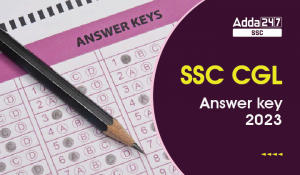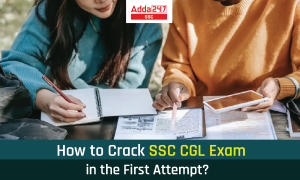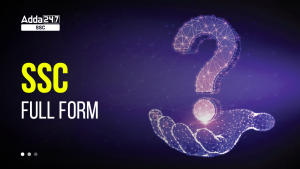Latest SSC jobs   »   Number System SSC CGL [Beginner level]...

# Number System SSC CGL [Beginner level] : 8th November

SSC CGL 2019 Exam notification is out once again raise the bar of competition. All the four sections bag impeccable practice when it comes to SSC Exams. Considering the same, at ADDA247 we are prompted to make Daily Quant Quiz accessible to you, aiming at a cut above preparation of latest exam pattern based questions. You are asked to practice Daily Quant Quiz at SSCADDA which encapsulates the exam like environment facilitated with extraordinary content. To make headway in upcoming SSC CGL Exams, go the extra mile with our Daily Quant Quiz.(a) 621/134
(b) 721/134
(c) 521/134
(d) 421/134(a) 355
(b) 356
(c) 357
(d) 359

Q3. What is the unit digit of (327)513 × (999)647 × (414)624 × (342)812
(a) 8
(b) 9
(c) 10
(d) 11

Q4. What is the unit digit of the sum of First 181 whole number ?
(a) 4
(b) 6
(c) 5
(d) 0

Q5. What is the unit digit of 1679 × 1183 × 397 × 295 × 1991 × 923 is ?
(a) 6
(b) 4
(c) 5
(d) 0

Q6. Find the last digit of the Expression 24 + 44 + 64 + 84 +_ _ _ _ 1824?
(a) 1
(b) 2
(c) 3
(d) 4

Q7. 4767 exactly divides ***341, The missing digits are
(a) 468
(b) 363
(c) 386
(d) 586

Q8. A number divided by 68 gives the quotient 269 and remainder zero. If the same number is divided by 67, then the remainder is:
(a) 0
(b) 1
(c) 2
(d) 3

Q9. The sum of the digits of a two-digit number is 81 less than the number. What is the difference between the digits of the number?
(a) 6
(b) 3
(c) 1
(d) Cannot be determined

Q10. If the digits of a two-digit number are interchanged, the number so obtained is greater than the original number by 27. If the sum of two digits of the number is 11, what is the original number?
(a) 47
(b) 38
(c) 35
(d) 49

#### solutions:-S5. Ans.(c)
Sol. Unit digit = 5
When 5 is multiplied with any odd number the unit digit will be 5

S7.Ans(d)
Sol.
Last digit of dividend = 1
Last digit of divisor = 7
Last digit of quotient should b 3
4767 × 3 = 14301
4767 × 20 = 95340
4767 × 100 = 476700
4767 × (3 + 20 + 100) = 586341
Missing digit are = 586

S8.Ans(b)
Sol
The number is 68 × 269 = 18292. 18292, when divided by 67, leaves a remainder of 1.

S9.Ans(d)
Sol.
10x + y – (x + y) = 81
or, 10x + y – x – y = 81
or, 9x ⇒ 81 ∴ x = 9
Hence, all such numbers are as follows: 90, 91, 92, 93, … 99.#### Congratulations!General Awareness & Science Capsule PDFIncorrect details? Fill the form again here

General Awareness & Science Capsule PDF

Thank You, Your details have been submitted we will get back to you.

### TOPICS:

•SSC CGL Marks 2023 Out, Scorecard Downlo...
•SSC CGL Final Answer Key 2023 Out: Tier ...
•SSC CGL Previous Year Question Paper Wit...
•How to Crack SSC CGL Exam in the First A...
•SSC CGL Nomination List, Check Region-wi...
•SSC Full Form: Staff Selection Commissio...